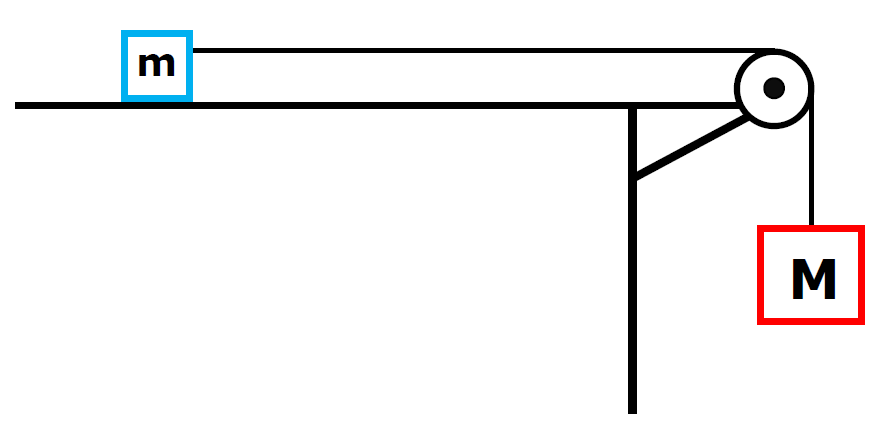﻿ Home`Find a for this system`
``
` `
```Two bodies move
as one mass w/ one accel.```
` `
``
`Fnet = ma`
`Let's make clockwise +`
`Mg = (m + M)a`
```The 2 masses get their accel.

from hanging mass```
` `
` `
`Applies only for frictionless surface`
 Mg = a (M+m)
` `
` `
` `

What would the accel. be if
the little mass was hanging?

` `
` `
` `
` `
 mg = a (M+m)
` `
`What is the tension force of rope?`
` `
`SFx = ma`
` `
`Tf = ma`
`Rule - One Rope, One Tension`
``
`Let's make clockwise +`
` `
` `
`Tension in terms of M`
``
 `ΣFy=Ma` ` ` `Mg - Tf = Ma ` ` ` `Mg - Ma = Tf `
` Tf  = M(g - a)  `
` `
` `
``
```If Box on table
`coefficient of friction = μ`
` `
``